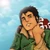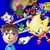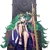24,862 Pages

## Overview

An earthquake (also known as a quake, tremor or temblor) is the perceptible shaking of the surface of the Earth. Earthquakes can be violent enough to toss people around and destroy whole cities. Such earthquakes being caused by characters are a method useable to determine Attack Potency.

For the purpose of these calculations shaking a minor area should not be assumed to automatically translate to an earthquake. Either a large area being shaken or evidence that we are dealing with something earthquake-like and not just some minor shaking would be needed.

One has to differentiate between different ways an earthquake was caused, if one wants to calculate the energy.

## Case 1: Meteor impact

This is the easiest method to determine the necessary energy to cause an earthquake, even though not the most commonly used one.

### In which cases should it be used?

This method should be used if an earthquake is caused through the impact of a meteor or through an event very similar to that.

### How is it done?

#### Step 1:

First step is to determine the distance of a place, for which we know which effects the earthquake had on, to the epicenter. To clarify: The epicenter is the point on the Earth's surface that is directly above the hypocenter or focus, the point where an earthquake or underground explosion originates. From the rest this calculator will be used.

#### Step 2:

Input the distance from the epicenter, which was determined in Step 1, in the "Distance from Impact" field.

After that input the rest of the values, for now it doesn't matter what stands in the rest of the fields.

Press "calculate effects".

If the inputs were sufficiently high on the page that appears will be a section named "Seismic effects".

In that section descriptions are given which describe the effects of an earthquake, which was caused by a meteor with the properties described by the prior input. Specifically this are the effects at the input distance from the epicenter.

All possible descriptions are listed in Table 1. What one now wants to do is to change the input values, except the "Distance from Impact" value, so long until you have the lowest values, for which still the description best fitting for the effects at your chosen distance from the epicenter is shown.

#### Step 3:

If you have found this values take a look at the "Energy" section above the "Seismic Effects" section on the page. The value given for "Energy before atmospheric entry" is the energy of your (theoretical) meteor and with that also the energy of necessary to cause the earthquake.

## Case 2: Natural Earthquakes

This method doesn't find much use, but is worth mentioning.

### In which cases should it be used?

This method should only be used in case a natural earthquake occurs, as it takes into account the work necessary to overcome the friction between continent plates etc.

It should be noted that, realistically speaking, a natural earthquake can't have a magnitude above about 9.6. So if the righter scale magnitude calculated is above that, this methology should only be used if special circumstances apply.

### How is it done?

First perform step 1 and step 2 like described in "Case 1: Meteor Impacts".

Since here one does not wish to know the energy necessary for a meteor, but for a real earthquake this time the third step has to be different.

Instead of looking at the "Energy" section, one looks at the "Seismic Effects" section again. There a value named "Richter Scale Magnitude" will be listed. This is the magnitude an earthquake would need to have to cause the described effects at the distance from the epicenter we input. The value is independent from the method through which the earthquake was caused, so it also stays true for other natural earthquakes which aren't caused by meteor impacts.

One takes the Richter Scale Magnitude and inputs it in this calculator in the "Earthquake Magnitude" field. Then press the compute button.

The value for "Total "Seismic Moment Energy" (MO)" is the energy necessary to cause such an earthquake.

## Case 3: Other

This is the most common method to calculate earthquakes.

### In which cases should it be used?

This should be used if the earthquake is not caused through something very similar to a meteor impact and is also not a natural earthquake.

### How is it done?

The method is identical to that of "Case 2: Natural Earthquakes", just this time instead of the "Total Seismic Moment Energy" (MO)" value our end result is the "Seismic Energy in Waves Radiated from Earthquake Source" value.

The reason one uses that value in this case is that the big energy loss that comes through the movement of the continental plate or similar processes of natural earthquakes is not present here. Instead we can only use the amount of energy that actually takes part in the effects we observer. Hence only the energy radiated in form of seismic waves is relevant for this case.

## Alternative Method to determine Richter Magnitude

A more direct method to figuring out the result is the following.

### 1. Mercalli Intensity to Magnitude at distance

The first step is the figure out the (Richter) Magnitude the earthquake corresponds to at the distance from the epicenter (origin) it is felt at. To do that we look at Table 1 above and pick the appropiate Intensity. Then we use that intensity to pick the appropiate "Magnitude at distance" from the table below.

Magnitude at distance Mercalli Intensity
1-2 I
2-3 I-II
3-4 III-IV
4-5 IV-V
5-6 VI-VII
6-7 VII-VIII
7-8 IX-X
8-9 X-XI
9+ XII

### 2. Magnitude at distance to Magnitude at origin

To do this knowing how far away from the origin we measured the Magnitude at distance is necessary. We call that distance r (unit should be km). To figure out how much the actual magnitude of the earthquake is we differentiate between 3 cases.

1. r < 60km: In this case the formula is (Magnitude at distance) + 0.0238*r = Richter Magnitude of Earthquake
2. 60 ≤ r < 700 km: In this case the formula is (Magnitude at distance) + 1.1644 + 0.0048*r = Richter Magnitude of Earthquake
3. r ≥ 700 km: In this case the formula is (Magnitude at distance) + 6.399 + 1.66*log10((r/110)*((2*π)/360)) = Richter Magnitude of Earthquake

### 3. Richter Magnitude to Energy

To get the energy from the Richter Magnitude we differentiate between 3 cases on how the earthquake was created:

1. Meteor Impact: The formula is 10(Richter Magnitude + 5.87)/0.67 = Energy in Joules
2. Real Earthquake: Just use this calculator. The Total Seismic Moment Energy is the result.
3. Others: Used for any artificially created earthquakes or earthquakes that aren't the former two. The formula is 101.5*(Richter Magnitude)+4.8 = Energy in Joules

## Start a Discussion Discussions about Earthquake Calculations

•#### Earthquake / Tsunami Calcs Revision

41 messages
•Migue79 wrote: I have now created a [https://vsbattles.fandom.com/wiki/User_blog:Migue79/Tsunami_via_Earthquake_feats blog] for the sm...
•The Calaca wrote: There's also Liger686 who made a 2020-02-13T12:39:26Z
•#### Problem with Earthquake calcs

108 messages
•Yeah, this thread can be closed now.
•I will close this thread then.
Community content is available under CC-BY-SA unless otherwise noted.